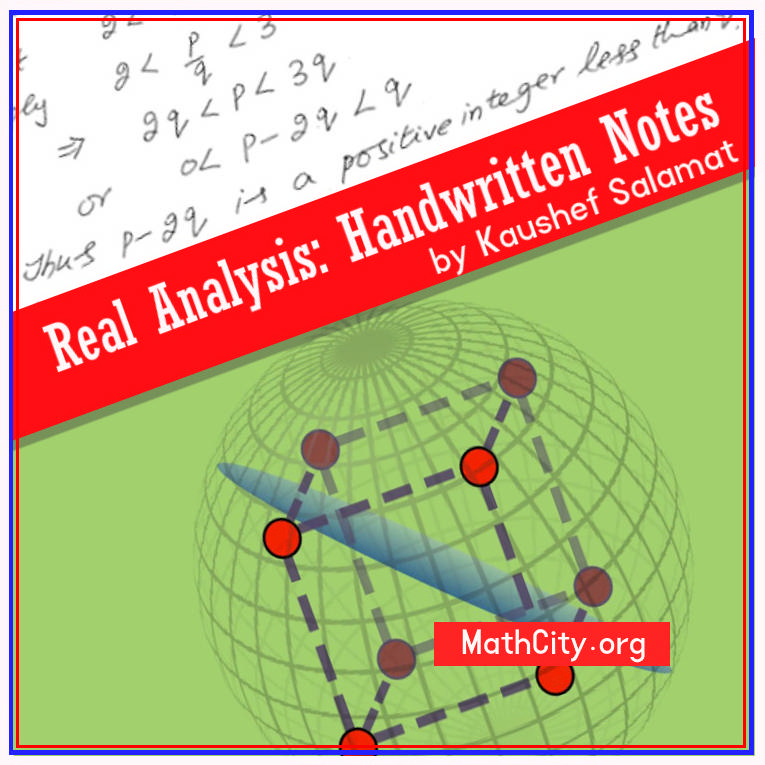# Real Analysis Handwritten Notes by Kaushef SalamatWe are very thankful to Ms. Kaushef Salamat for providing these notes. Real Analysis is a core subject in BS or MSc Mathematics. Without a fundamental grasp of Real Analysis, one cannot claim to be a mathematician. These notes are very comprehensive containing almost all the notions of Real Analysis. For providing these notes, Ms. Iqra Liaqat has our sincere gratitude.

 Name Real Analysis: Handwritten Notes Kaushef Salamat 344 pages PDF (see Software section for PDF Reader) 8.52 MB
• Introduce Sets
• Methods of Proof
• Contrapositive
• Terminology
• Ordered Set
• Ordered Field
• Bounded and Unbounded Sets
• Archimedian Principle
• Condensation Property
• The Extended Real Number System
• Absolute Value of a Real Number
• Schwarz Inequality
• Euclidean Space
• Inner Product
• Norm
• Dedekind's Property
• Inclusion Function
• Inverse Function
• Metric
• Open Cover
• Compact Set
• Open Cover
• Separable Sets
• Disconnected
• Sequences
• Monotone Sequences
• Euler Number
• Subsequences
• Urysohn Property
• Monotone Subsequence Theorem
• The Bolzano-weirstrass Theorem
• Cauchy Sequence
• Contractive Sequence
• Properly Divergent Sequence
• Infinite Limits
• Oscillate Sequence
• Properly Divergent
• Comparison Theorem
• Limit Inferior and Limit Superior
• Cluster Point
• Cauchy's Second theorem on Limit
• Sets of Real Numbers
• Heine-Borel (Covering) Theorem
• Infinite Series
• Cauchy Criterion
• Consequence of Cauchy Criterion
• Comparison Test
• Limit Comparison Test
• Absolute and Conditionally Convergent Series
• Rearrangement of Series
• Test for Absolute Convergence, Cauchy Root Test
• Ratio Test, Raabe's Test, Bertrand's Test
• Guass's Test, First Log Test, Second Log Test
• Alternating Series
• Abel's Lemma
• Dirichlet Test
• Abel's test
• Limits (Limits of Functions)
• Limits of Function at a Real Number
• Sequential Criterion for Limits
• Divergence Criteria
• Bounded Functions
• Sequeeze (Sandwich) Theorem
• Some Extensions of the Limit Concepts
• Monotone Function
• Continuous Functions
• Composition of Continuous Functions
• Properties of Continuous Function
• Extreme Value Theorem
• Bolzano's Intermediate Value Theorem
• Preservation of Intervals Theorem
• Brouwer's Fixed Point Theorem
• Continuous Inverse Theorem
• Uniform Continuity
• Continuous Extension Theorem
• Pecewise Linear Function
• Differentiation
• Chain Rule
• Inverse Function
• Darboux's Theorem
• Criterion for Integrability
• Improper Integrals
• Beta Function
• Absolute Convergence
• Infinite Range of Integration
• Comparison Test for Convergence at $\infty$

• notes/real-analysis-hand-written-kaushef